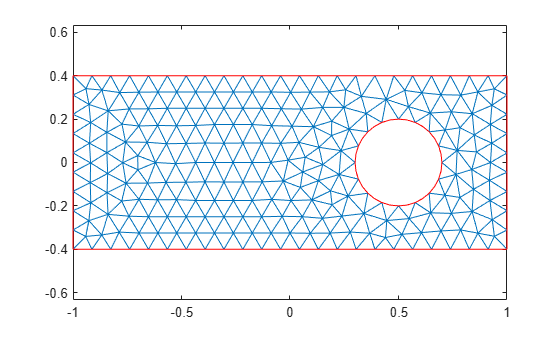# meshToPet

`[p,e,t]` representation of `FEMesh` data

This page describes the legacy workflow. New features might not be compatible with the `[p,e,t]` representation of `FEMesh` data.

## Syntax

``````[p,e,t] = meshToPet(mesh)``````

## Description

example

``````[p,e,t] = meshToPet(mesh)``` extracts the legacy `[p,e,t]` mesh representation from a FEMesh Properties object.```

## Examples

collapse all

This example shows how to convert a mesh in object form to `[p,e,t]` form.

Create a 2-D PDE geometry and incorporate it into a model object. View the geometry.

```model = createpde(1); R1 = [3,4,-1,1,1,-1,-.4,-.4,.4,.4]'; C1 = [1,.5,0,.2]'; % Pad C1 with zeros to enable concatenation with R1 C1 = [C1;zeros(length(R1)-length(C1),1)]; geom = [R1,C1]; ns = (char('R1','C1'))'; sf = 'R1-C1'; gd = decsg(geom,sf,ns); geometryFromEdges(model,gd); pdegplot(model,'EdgeLabels','on') xlim([-1.1 1.1]) axis equal```Create a mesh for the geometry. View the mesh.

```generateMesh(model); pdemesh(model) axis equal```Convert the mesh to `[p,e,t]` form.

`[p,e,t] = meshToPet(model.Mesh);`

View the sizes of the `[p,e,t]` matrices.

`size(p)`
```ans = 1×2 2 956 ```
`size(e)`
```ans = 1×2 7 160 ```
`size(t)`
```ans = 1×2 7 438 ```

## Input Arguments

collapse all

Mesh object, specified as the `Mesh` property of a `PDEModel` object or as the output of `generateMesh`.

Example: `model.Mesh`

## Output Arguments

collapse all

Mesh points, returned as a 2-by-`Np` matrix (2-D geometry) or a 3-by-`Np` matrix (3-D geometry). `Np` is the number of points (nodes) in the mesh. Column `k` of `p` consists of the x-coordinate of point `k` in `p(1,k)`, the y-coordinate of point `k` in `p(2,k)`, and, for 3-D, the z-coordinate of point `k` in `p(3,k)`. For details, see Mesh Data.

Mesh edges, returned as a 7-by-`Ne` matrix (2-D), or a mesh associativity object (3-D). `Ne` is the number of edges in the mesh. An edge is a pair of points in `p` containing a boundary between subdomains, or containing an outer boundary. For details, see Mesh Data.

Mesh elements, returned as a 4-by-`Nt` matrix (2-D with linear elements), a 7-by-`Nt` matrix (2-D with quadratic elements), a 5-by-`Nt` matrix (3-D with linear elements), or an 11-by-`Nt` matrix (3-D with quadratic elements). `Nt` is the number of triangles or tetrahedra in the mesh.

The `t(i,k)`, with `i` ranging from 1 through `end - 1`, contain indices to the corner points and possibly edge centers of element `k`. For details, see Mesh Data. The last row, `t(end,k)`, contains the subdomain number of the element.

## Version History

Introduced in R2015a